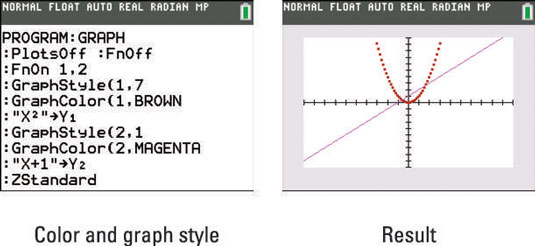##### TI-84 Plus CE Graphing Calculator For Dummies, 3rd EditionWith the TI-84 Plus C calculator, you can change the color as well as the graph style of functions on the TI-84 Plus. In this program, the GraphStyle(function#,graph style#) command is used to change the attributes of a function. Entering a function number of 2 changes the graph style of function Y2.There are eight different graph styles: 1=thin, 2=thick, 3=above, 4=below, 5=path, 6=animate, 7=dot-thick, 8=dot-thin.

The GraphColor(function#,color#) command changes the color of a function. There are 15 colors to choose from: 10=Blue, 11=Red, 12=Black, 13=Magenta, 14=Green, 15=Orange, 16=Brown, 17=Navy, 18=LtBlue, 19=Yellow, 20=White, 21=LtGray, 22=MedGray, 23=Gray, and 24=DarkGray.

When using the Graph Color command, you may enter its corresponding number or press [VARS] and the left-arrow key to access the Vars COLOR menu and make a color selection. Of course, the Graph Style and Graph Color commands should be used prior to graphing the function.It is OK to leave off the right parenthesis at the end of a command in a program, because the program will take up less RAM on the calculator.